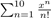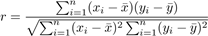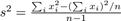# We Helped With This R Language Programming Assignment: Have A Similar One?

SOLVEDCategory Programming R | R Studio Undergraduate Solved Probability Homework Help

## Short Assignment Requirements

A 10 problem homework assignment on basic R programming.

## Assignment Description

1### Stat 471/671, Spring 2019, Hwk 1, due Feb 14, 11:00am

Q1. Use the rep() function (once for each part) to generate the following vectors:

1)   1 1 1 1 1 1 1 2 2 2 2 2 2 2 3 3 3 3 3 3 3

2)   1 1 1 1 1 1 2 2 2 2 2 3 3 3 3 4 4 4 5 5 6

3)   1 1 1 1 1 2 3 3 3 4 4 4 4 5 5

Q2. Let x = y = (0,−3,NA,13)0. Without help of the function identical(), check whether or not x = y.

Q3. Use vectorization to evaluatefor x = 5 in a single R expression without using loops or writing out the summation one term at a time. You may use the R function factorial().

Q4. Write single R expressions to do the following. For the numbers

7.5, 6.9, 0.06, 0.006, 12, 2.7, 17, 8, 0.8, 6.9,

compute

(a)    the square roots of the absolute deviations of the numbers from their mean.

(b)    the largest of the squared deviations of the numbers from their median.

(c)    the absolute errors due to rounding: Round the square roots of the numbers totwo decimal places and square them. Find the absolute differences between these and the original numbers.

Q5. Write a single R expression to calculate the sample correlation coefficient r between the two variables: Age and Circumference from the Orange data set using the formulaYou may not use the R functions cov() or var() for writing this expression. Use the cor() function to check your answer.

Q6. Use a single R expression and the the sample variance formulato calculate the sample variance of the weight in the chickwts data set.

Q7. Using a single R expression, calculate the sample median absolute deviation (mad)

1.4826 × med|xi − med(xi)|

of the weight in the chickwts data set, where med(xi) is the sample median and med|xi− med(xi)| is the sample median of the absolute deviations of xi from med(xi).

Q8. Create an R matrix object named A using the following data:

24.5 -2.1 6.3 2.7 1.6 -2.6 3.6 8.4 5.2 8.3

2.5 1.7 3.7 -4.4 3.7

3.8 5.8 5.2 1.9 -4.2

Do this by using the scan() function to read in the data from screen. Perform the following operations and report the result of each operation:

(a)    extract the middle 3 elements of row 3 of A to a vector y;

(b)    create the following 3 × 3 matrix B by subsetting matrix A:

3.6 5.2 8.3

1.7 -4.4 3.7

5.8 1.9 -4.2

(c)    compute vector d = By;

(d)   append the data vector (9.4,10.5,−11.5)0 to the left hand side of B to form W;

(e)    compute matrix P = WW0;

(f)     compute the inverse of P;

(g)    solve the equation Pa = d for a using a = P−1d;

(h)    solve the equation Pa = d for a using function solve()

(i)     factorize P so that P = R0R, where R is an upper triangular matrix.

Q9. Use the matrix A created in Q8 to write a single expression to extract

(a)    the row of A that contains the largest value of the matrix;

(b)    the column of A that contains the smallest value of the matrix.

Q10. Elements of an R data frame can also be accessed the same way elements of a matrix are accessed using subscripts. Subsets of a data frame extracted this way will themselves be data frames, except when they are single rows, columns, or scalars. In general, a single row will be a data frame and a single column, an R vector.

Load the package named MASS and the Cars93 data set available in the package using data(Cars93, package="MASS"). Use subscripting operations on Cars93 to find the vehicles with highway mileage of less than 25 miles per gallon (variable MPG.highway) and weight (variable Weight) over 3500 lbs. Report the model name, the price range (low, high), highway mileage, and the weight of the cars that satisfy these conditions. This can be done in a single R expression; however, you may simplify the extraction to several steps.

Is it free to get my assignment evaluated?

Yes. No hidden fees. You pay for the solution only, and all the explanations about how to run it are included in the price. It takes up to 24 hours to get a quote from an expert. In some cases, we can help you faster if an expert is available, but you should always order in advance to avoid the risks. You can place a new order here.

How much does it cost?

The cost depends on many factors: how far away the deadline is, how hard/big the task is, if it is code only or a report, etc. We try to give rough estimates here, but it is just for orientation (in USD):

 Regular homework \$20 - \$150 Advanced homework \$100 - \$300 Group project or a report \$200 - \$500 Mid-term or final project \$200 - \$800 Live exam help \$100 - \$300 Full thesis \$1000 - \$3000

How do I pay?

Credit card or PayPal. You don't need to create/have a Payal account in order to pay by a credit card. Paypal offers you "buyer's protection" in case of any issues.

Why do I need to pay in advance?

We have no way to request money after we send you the solution. PayPal works as a middleman, which protects you in case of any disputes, so you should feel safe paying using PayPal.

Do you do essays?

No, unless it is a data analysis essay or report. This is because essays are very personal and it is easy to see when they are written by another person. This is not the case with math and programming.

Why there are no discounts?

It is because we don't want to lie - in such services no discount can be set in advance because we set the price knowing that there is a discount. For example, if we wanted to ask for \$100, we could tell that the price is \$200 and because you are special, we can do a 50% discount. It is the way all scam websites operate. We set honest prices instead, so there is no need for fake discounts.

Do you do live tutoring?

No, it is simply not how we operate. How often do you meet a great programmer who is also a great speaker? Rarely. It is why we encourage our experts to write down explanations instead of having a live call. It is often enough to get you started - analyzing and running the solutions is a big part of learning.

What happens if I am not satisfied with the solution?

Another expert will review the task, and if your claim is reasonable - we refund the payment and often block the freelancer from our platform. Because we are so harsh with our experts - the ones working with us are very trustworthy to deliver high-quality assignment solutions on time.

## Popular Solved Assignments Like This

Customer Feedback

"Thanks for explanations after the assignment was already completed... Emily is such a nice tutor! "

Order #13073